Roman Numerals 1
Set 1 (10 Questions)
Students learn the value of the various Roman Numerals. Students compare the value of Roman and standard numerals. Students have access to links about the historical use of Roman Numerals.
From Mr. Anker Tests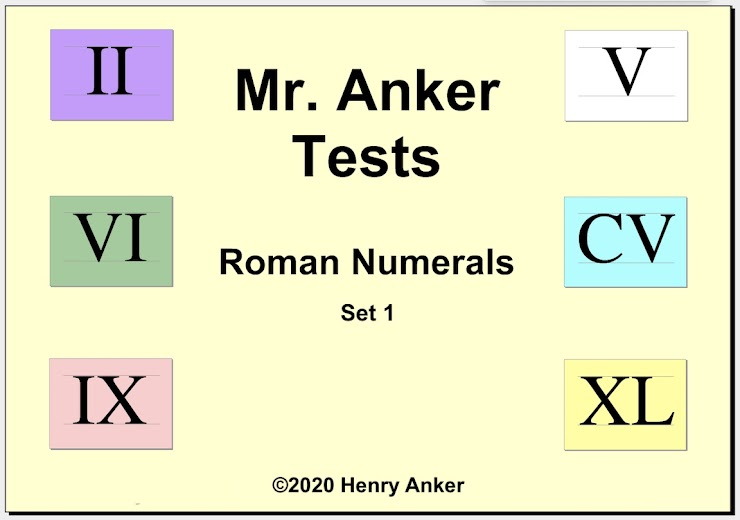Roman Numerals Help Slide 1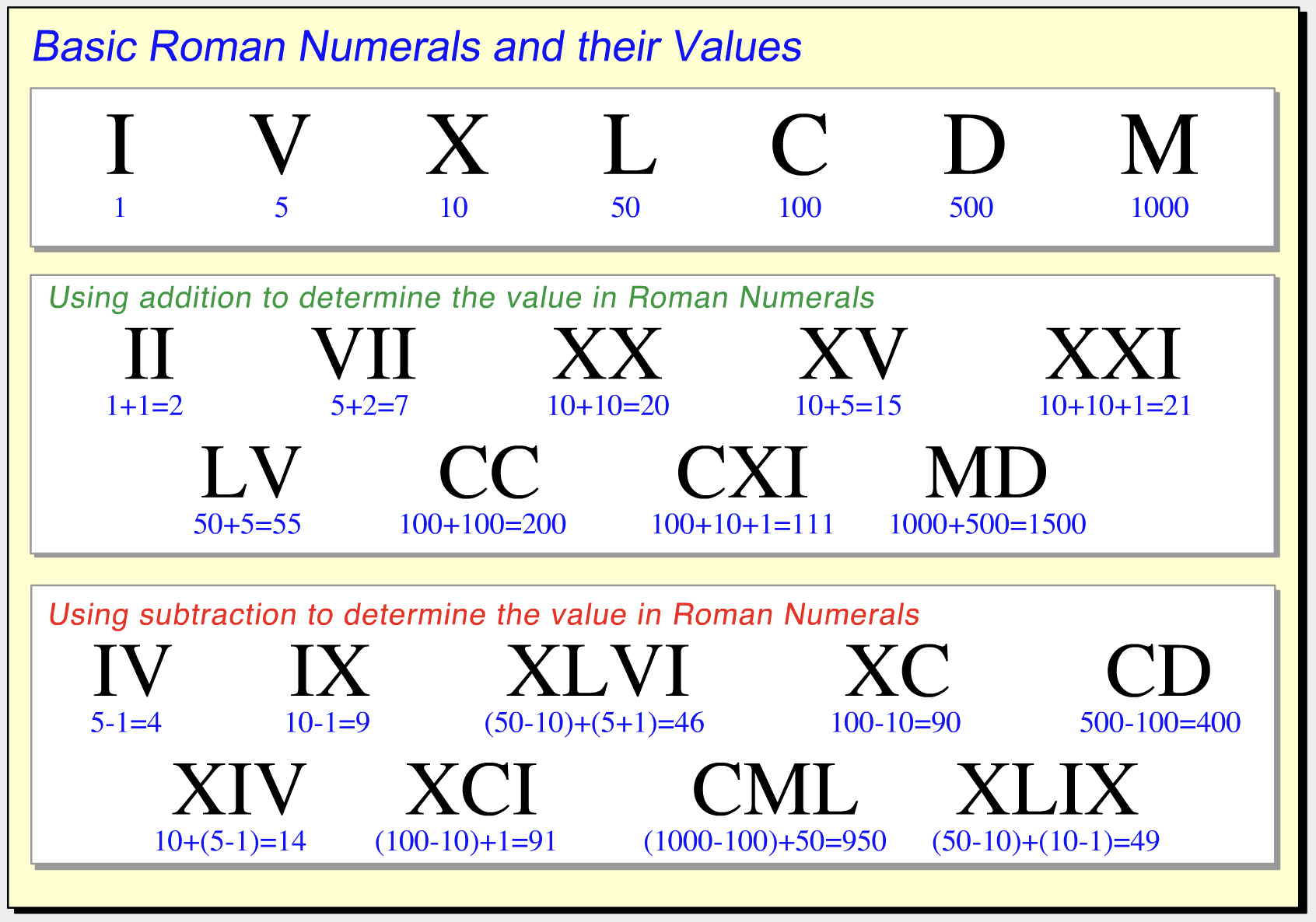This outstanding video can be found on Socratica Math
Roman Numerals Help Slide 2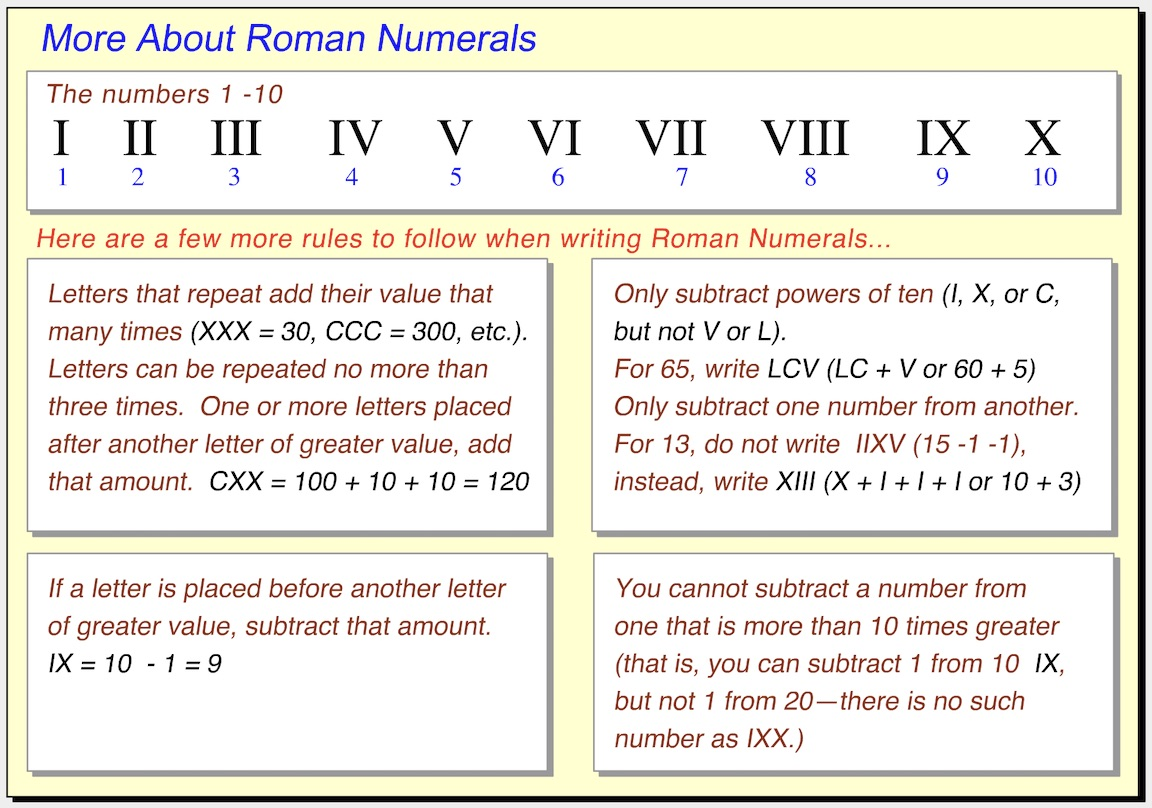1.  Match the standard numeral to this Roman Numeral. *
1 point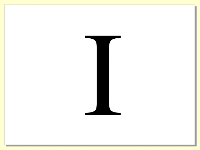2.  Match the standard numeral to this Roman Numeral. *
1 point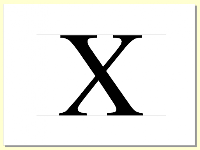3.  Match the standard numeral to this Roman Numeral. *
1 point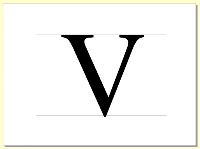4.  Match the standard numeral to this Roman Numeral. *
1 point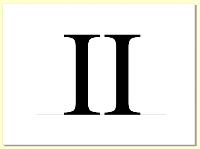5.  Match the standard numeral to this Roman Numeral. *
1 point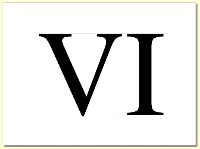6.  Match the Roman Numeral to this standard numeral. *
1 point7.  Match the Roman Numeral to this standard numeral. *
1 point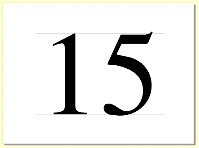8.  Match the Roman Numeral to this standard numeral. *
1 point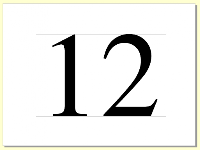9.  Match the Roman Numeral to this standard numeral. *
1 point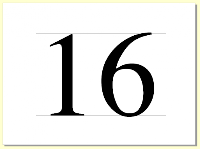10.  Match the Roman Numeral to this standard numeral. *
1 point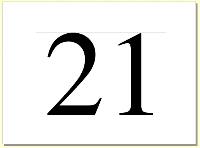Submit
Clear form